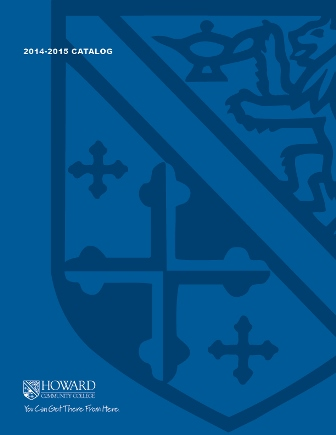2017-2018 College Catalog# MATH 067 Elementary Algebra

Students will develop skills in simplifying polynomials, rational expressions, and radicals. Methods of factoring second-degree polynomials and applications involving factoring will also be included. Students will solve quadratic equations and related applications using factoring and the quadratic formula. They will become familiar with triangles, midpoint, and distance between two points. Students will use ratios and proportions to solve various problems. Application problems will include the use of the Theorem of Pythagoras and scientific notation. All sections require the use of the interactive computer program that comes with the software code. Use of a calculator is limited to a four-function calculator.

4

### Prerequisite

An appropriate score on the mathematics placement test, or a grade of B or higher in MATH 061 or a grade of C in MATH 063

FYEX 100

### Hours Weekly

4 hours computer-based instructional lab, 1.5 hours recitation weekly

### Course Objectives

1. 1. Multiply and divide polynomials.
2. 2. Factor second-degree polynomials.
3. 3. Combine and simplify expressions involving radicals.
4. 4. Solve quadratic equations by factoring and the square root method or by applying the
5. 5. Multiply and divide polynomial rational expressions.
6. 6. Convert numbers between standard and scientific notation.
7. 7. Apply geometric principles to solve problems involving triangles using the rules of similar
triangles or the Pythagorean Theorem.
8. 8. Solve problems involving midpoint and distance between two points.
9. 9. Solve proportion problems using the Means/Extremes Product Property.
10. 10. Solve applied problems involving direct and inverse variation.
11. 11. Develop skills needed to use technology appropriately as a learning tool.

### Course Objectives

1. Multiply and divide polynomials.

2. Factor second-degree polynomials.

3. Combine and simplify expressions involving radicals.

4. Solve quadratic equations by factoring and the square root method or by applying the

5. Multiply and divide polynomial rational expressions.

6. Convert numbers between standard and scientific notation.

7. Apply geometric principles to solve problems involving triangles using the rules of similar
triangles or the Pythagorean Theorem.

8. Solve problems involving midpoint and distance between two points.

9. Solve proportion problems using the Means/Extremes Product Property.

10. Solve applied problems involving direct and inverse variation.

11. Develop skills needed to use technology appropriately as a learning tool.